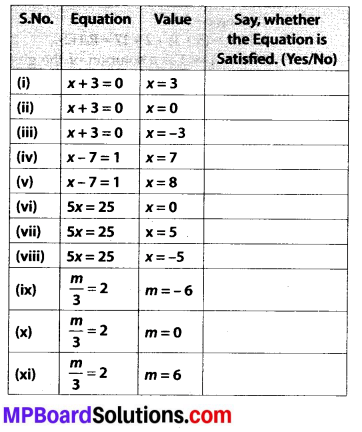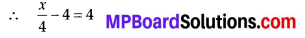# MP Board Class 7th Maths Solutions Chapter 4 Simple Equations Ex 4.1

## MP Board Class 7th Maths Solutions Chapter 4 Simple Equations Ex 4.1

Question 1.
Complete the last column of the table.Solution:
(i) x + 3 = 0
By putting x = 3
L.H.S. = 3 + 3 = 6 ≠ R.H.S.
Hence, the equation is not satisfied.

(ii) x + 3 = 0
By putting x = 0
L.H.S. = 0 + 3 = 3 ≠ R.H.S.
Hence, the equation is not satisfied.

(iii) x + 3 = 0
By putting x = – 3
L.H.S. = -3 + 3 = 0 = R.H.S.
Hence, the equation is satisfied.

(iv) x – 7 = 1
By putting x = 7
L.H.S. = 7 – 7 = 0 ≠ R.H.S.
Hence, the equation is not satisfied.

(v) x – 7 = 1
By putting x = 8 L.H.S. = 8 – 7 = 1 = R.H.S.
Hence, the equation is satisfied.

(vi) 5x = 25
By putting x = 0
L.H.S. = 5 × 0 = 0 ≠ R.H.S.
Hence, the equation is not satisfied.

(vii) 5x = 25
By putting x = 5
L.H.S. = 5 × 5 = 25 = R.H.S.
Hence, the equation is satisfied,

(viii) 5x = 25 .
By putting x = – 5
L.H.S. = 5 × (- 5) = – 25 ≠ R.H.S.
Hence, the equation is not satisfied.

(ix) $$\frac{m}{3}$$ = 2
By putting m = – 6
L.H.S. = $$\frac{-6}{3}$$ = -2 ≠ R.H.S.
Hence, the equation is not satisfied.

(x) $$\frac{m}{3}$$ = 2
By putting m = 0
L.H.S. = $$\frac{0}{3}$$ = 0 ≠ R.H.S.
Hence, the equation is not satisfied.

(xi) $$\frac{m}{3}$$ = 3
By putting m = 6
L.H.S. = $$\frac{6}{3}$$ = 2 = R.H.S
Hence, the equation is satisfied.Question 2.
Check whether the value given in the brackets is a solution to the given equation or not:
(a) n + 5 = 19(n = 1)
(b) 7n + 5 = 19(n = -2)
(c) 7n + 5 = 19(n = 2)
(d) 4p – 3 = 13(p = 1)
(e) 4p – 3 = 13(p = -4)
(f) 4p – 3 = 13 (p = 0)
Solution:
(a) n + 5 = 19 (n = 1)
Putting n = 1
L.H.S. = n + 5 = 1 + 5 = 6 ≠ 19 = R.H.S.
As L.H.S. ≠ R.H.S.
Therefore, n = 1 is not a solution of the given equation, n + 5 = 19.

(b) 7n + 5 = 19 (n =- 2)
Putting n = – 2
L.H.S. = 7n + 5 = 7 × (-2) + 5 = -14 + 5
= -9 ≠ 19 = R.H.S.
As L.H.S. ≠ R.H.S.
Therefore, n = -2 is not a solution of the given equation, 7n + 5 = 19.

(c) 7n + 5 = 19 (n = 2)
Putting n = 2
L.HS. = 7n + 5 = 7 × (2) + 5 = 14 + 5 = 19 = R.HS.
As L.H.S. = R.H.S.
Therefore, n = 2 is a solution of the given equation, 7n + 5 = 19.

(d) 4p – 3 = 13(p = 1)
Putting p = 1
L.H.S. = 4p – 3 = (4 × 1) – 3
= 1 × 13 = R.H.S.
As L.H.S. × R.H.S.
Therefore, p = 1 is not a solution of the given equation, 4p – 3 = 13.

(e) 4p – 3 = 13 (p = – 4)
Putting p = – 4
L.H.S. = 4p – 3 = 4 × (- 4) – 3 = -16 – 3
= -19 ≠ 13 = R.H.S.
As L.H.S. ≠ R.H.S.
Therefore, p = – 4 is not a solution of the given equation, 4p – 3 = 13.

(f) 4p – 3 = 13 (p = 0)
Putting p = 0
L.HS. = 4p – 3 = (4 × 0) – 3 = -3 ≠ 13 = RH.S.
As L.H.S. ≠ R.H.S.
Therefore, p = 0 is not a solution of the given equation, 4p – 3 = 13.Question 3.
Solve the following equations by trial and error method:
(i) 5p + 2 = 17
(ii) 3m -14 = 4
Solution:
(i) 5p + 2 = 17 Putting p = 1
L.H.S. = (5 × 1)+ 2 = 7 ≠ R.H.S.
Putting p = 2
L.H.S. = (5 × 2) + 2 = 10 + 2 = 12 ≠ R.H.S.
Putting p = 3
L.H.S. = (5 × 3) + 2 = 17 = R.H.S.
Hence, p = 3 is a solution of the given equation.

(ii) 3m – 14 = 4
Putting m = 4,
L.H.S. = (3 × 4) – 14 = -2 ≠ R.H.S.
Putting m = 5,
L.H.S. = (3 × 5) – 14 = 1 ≠ R.H.S.
Putting m = 6,
L.H.S. = (3 × 6) – 14 = 18 -14 = 4 = R.H.S.
Hence, m = 6 is a solution of the given equation.Question 4.
Write equations for the following statements:
(i) The sum of numbers x and 4 is 9.
(ii) 2 subtracted from y is 8.
(iii) Ten times a is 70.
(iv) The number b divided by 5 gives 6.
(v) Three-fourth of f is 15.
(vi) Seven times m plus 7 gives 77.
(vii) One-fourth of a number x minus 4 gives 4.
(viii) If you take away 6 from 6 times y, you get 60.
(ix) If you add 3 to one-third of z, you get 30.
Solution:
(i) x + 4 = 9
(ii) y – 2 = 8
(iii) 10a = 70
(iv) $$\frac{b}{5}$$ = 6
(v) $$\frac{3}{4} t$$ = 15
(vi) Seven times of m is 7m.
∴ 7m + 7 = 77
(vii) One-fourth of a number x is $$\frac{x}{4}$$.(viii) Six times of y is 6y.
∴ 6y – y = 60

(ix) One-third of z is $$\frac{x}{4}$$ .Question 5.
Write the following equations in statement forms:
(i) p + 4 = 15
(ii) m – 7 = 3
(iii) 2m = 7
(iv) $$\frac{m}{5}$$ = 3
(v) $$\frac{3 m}{5}$$ = 6
(vi) 3p + 4 = 25
(viii) $$\frac{p}{2}$$ + 2 = 8
Solution:
(i) The sum of p and 4 is 15.
(ii) 7 subtracted from m is 3.
(iii) Twice of a number m is 7.
(iv) One-fifth of a number m is 3.
(v) Three-fifth of a number m is 6.
(vi) Three times of a number p, when added to 4, gives 25.
(vii) 2 subtracted from four times of a number p is 18.
(viii) 2 added to half of a number p gives 8.Question 6.
Set up an equation in the following cases:
(i) Irfan says that he has 7 marbles more than five times the marbles Parmit has. Irfan has 37 marbles. (Take m to be the number of Parmit’s marbles.)
(ii) Laxmi’s father is 49 years old. He is 4 years older than three times Laxmi’s age. (Take Laxmi’s age to be y years.)
(iii) The teacher tells the class that the highest marks obtained by a student in her class is twice the lowest marks plus 7. The highest score is 87, (Take the lowest score to be l.)
(iv) In an isosceles triangle, the vertex angle is twice either base angle. (Let the base angle be b in degrees. Remember that the sum of angles of a triangle is 180 degrees.)
Solution:
(i) Let Parmit has in marbles.
Number of marbles Irfan has = 5 × Number of marbles Parmit has +7
∴ 5 × m + 7 = 37 ⇒ 5m + 7 = 37

(ii) Let Laxmi be i years old.
Laxmi’s father’s age 3 × Laxmi’s age +4
∴ 49 = 3xy + 4 ⇒ 3y + 4 = 49

(iii) Let the lowest marks be 1.
Highest marks = 2 × Lowest marks +7
∴ 87 = 2 × l + 7 ⇒ 2l + 7 = 87

(iv) An isosceles triangle has two of its angles of equal measure.
Let base angle be b.
Vertex angle =2 × Base angle = 2b
Sum of all interior angles of a triangle 180°
∴ b + b + 2b = 180° ⇒ 4b = 180°